# Finding the Area of Figures using Unit Squares: Grade 3 Math Lesson

Page content

Without overlapping or leaving gaps, cover the geometric figures (squares, rectangles, and irregular figures) with “unit squares”. To determine the area of geometric figures, count the number of “unit squares” used to cover the figures.

Lesson Objective: The lesson is aligned to the Common Core State Standards for Mathematics – 3.MD.5a and b Geometric Measurement – Recognize area as an attribute of plane figures and understand concepts of area measurement.

Materials Required: unit squares

## Lesson Procedure:

**

Identifying Area of a “Unit Square”

1. Identify the side length of the “unit square” as one unit.
2. Identify the area of the “unit square” as one square unit.
3. Use the “unit square” to measure the area of each of the geometric figures.
4. Trace the “unit square” twenty times and cut out each “unit square”.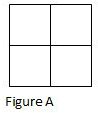Measuring Area of Geometric Figures

1. Without gaps or overlaps, cover Figure A with the “unit squares”.
2. Count the number of “unit squares” used to cover the figure.
3. How many “unit squares” were used to cover the figure?
4. What is the area, in square units, of Figure A?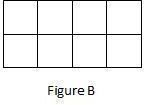5. Without gaps or overlaps, cover Figure B with the “unit squares”.
6. Count the number of “unit squares” used to cover the figure.
7. How many “unit squares” were used to cover the figure?
8. What is the area, in square units, of Figure B?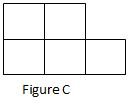9. Without gaps or overlaps, cover Figure C with the “unit squares”.
10. Count the number of “unit squares” used to cover the figure.
11. How many “unit squares” were used to cover the figure?
12. What is the area, in square units, of Figure C?

3. 4

4. 4 square units

7. 10

8. 10 square units

11. 5

12. 5 square units

## Individual or Group Work:

1.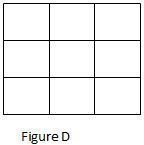Without gaps or overlaps, cover Figure D with the “unit squares”.

2. Count the number of “unit squares” used to cover the figure.

3. How many “unit squares” were used to cover the figure?

4. What is the area, in square units, of Figure D?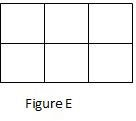5. Without gaps or overlaps, cover Figure E with the “unit squares”.
6. Count the number of “unit squares” used to cover the figure.
7. How many “unit squares” were used to cover the figure?
8. What is the area, in square units, of Figure E?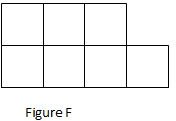9. Without gaps or overlaps, cover Figure F with the “unit squares”.
10. Count the number of “unit squares” used to cover the figure.
11. How many “unit squares” were used to cover the figure?
12. What is the area, in square units, of Figure F?

3. 9

4. square units

7. 6

8. 6 square units

11. 7

12. 7 square units

Students should be able to determine the area of geometric figures (squares, rectangles, and irregular figures) by counting the number of “unit squares” needed to cover the figures.